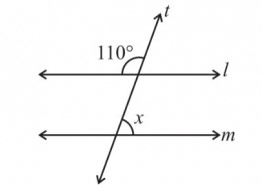"
">

# Find the value of x, if $l \parallel m$."

Given :

In the given figure, $l \parallel m$ and t is transversal.

One of the angles is 110°.

To do :

We have to find the value of x.

Solution :

It is given that, $l \parallel m$ and t is transversal.

We know that corresponding angles are equal.

x is the corresponding angle of the angle near to 110°.

Therefore, 110° and x are in a straight line.

So, $x +110° = 180°$

$x = 180° - 110° = 70°$

Therefore, the value of x is 70°.

Updated on: 10-Oct-2022

145 Views